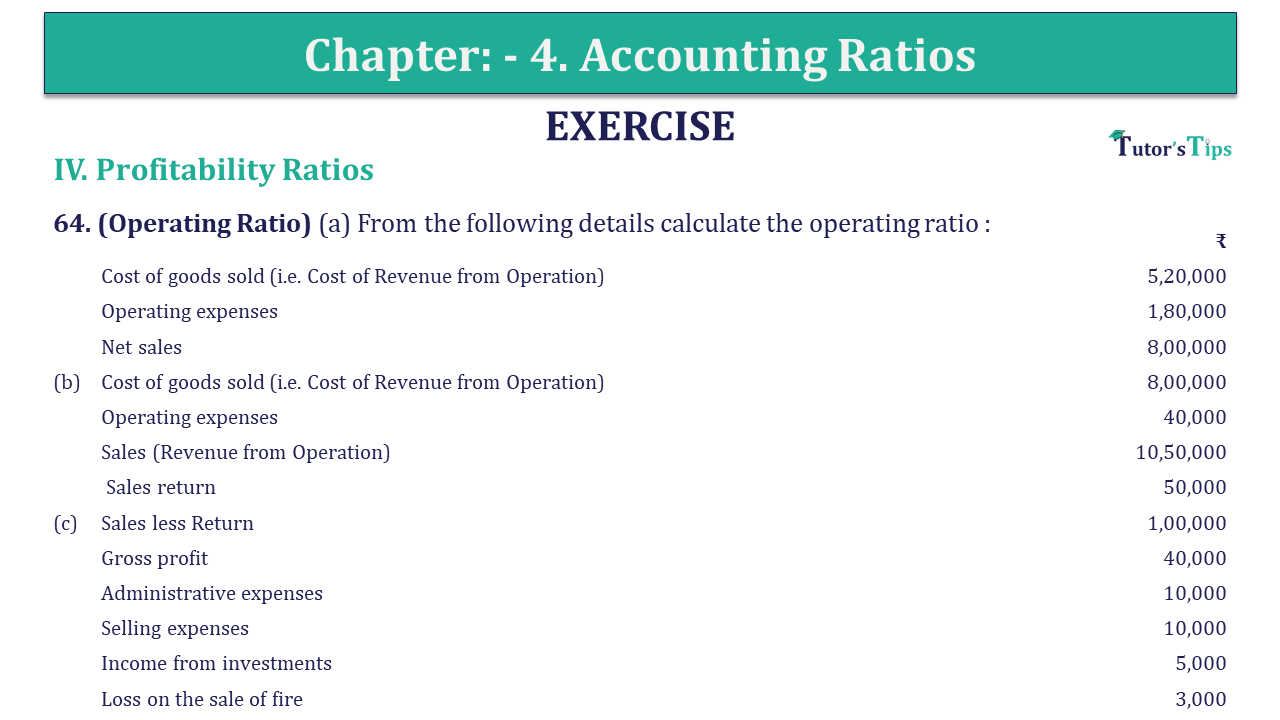# Question 64 Chapter 4 of +2-B – USHA Publication 12 ClassQ-64- CH-4 Book 2 - Usha Pub. +2 Book 2020 - Solution

Question 64 Chapter 4 of +2-B

Profitability Ratio

64. (Operating Ratio) (a) From the following details calculate the operating ratio :

 ₹ Cost of goods sold (i.e. Cost of Revenue from Operation) 5,20,000 Operating expenses 1,80,000 Net sales 8,00,000 (b) Cost of goods sold (i.e. Cost of Revenue from Operation) 8,00,000 Operating expenses 40,000 Sales (Revenue from Operation) 10,50,000 Sales return 50,000 (c) Sales less Return 1,00,000 Gross profit 40,000 Administrative expenses 10,000 Selling expenses 10,000 Income from investments 5,000 Loss on the sale of fire 3,000

## The solution of Question 64 Chapter 4 of +2-B: –

 (a) Operating Ratio = Operating Cost X 100 Net Sales = ₹ 7,00,000 X 100 ₹ 8,00,000 = 87.5% * Operating Cost = Cost of goods sold + Operating expenses = ₹ 5,20,000 – ₹ 1,80,000 = ₹ 7,00,000

 (b)Operating Ratio = ₹ 8,40,000 X 100 ₹ 10,00,000 = 84%
 *Operating Cost = Cost of goods sold + Operating expenses = ₹ 8,00,000 + ₹ 40,000 = ₹ 8,40,000 *Net Sales = Sales – Sales Return = ₹ 10,50,000 – ₹ 50,000 = ₹ 10,00,000
 (c)Operating Ratio = ₹ 80,000 X 100 ₹ 1,00,000 = 80%
 Cost of goods sold = Sales – Gross Profit = ₹ 1,00,000 + ₹ 40,000 = ₹ 60,000 Operating Cost = Cost of goods sold + Administrative expenses + Selling expenses = ₹ 60,000 + ₹ 10,000 + ₹ 10,000 = ₹ 80,000

Also, Check out the solved question of previous Chapters: –